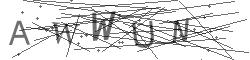# Current report Return >

## 2016

Number Date Subject
59/2016 2016-10-13 Notification on change of shares

The Management Board of Boryszew S.A. (the company) announces that the company received the

notification from Mr. Roman Krzysztof Karkosik informing that on 11 October, 2016 he got an

information from Boryszew S.A., that on the brokerage account of Impexmetal S.A. has been

transferred ownership 5,618,412 units of shares of Boryszew S.A., which constitutes 2,34% of equity,

giving right to 5,618,412 votes at the GM of Boryszew S.A., which are equal to 2.34% of the total

number of votes at the GM.

The above mentioned transaction has resulted in change of indirectly share of Mr. Roman Krzysztof

Karkosik in the total number of votes at GM of Boryszew S.A. more than 1 %.

On 9 October, 2016, i.e. before the transaction Mr. Roman Krzysztof Karkosik owned directly

127,330,873 units of shares of Boryszew S.A., which constituted 53.054% of equity and indirectly

through subsidiaries 19,200,000 units of shares of Boryszew S.A., which constituted 8.00% of equity.

The possessed shares have given directly 126,862,398 votes at the GM of Boryszew S.A., which are

equal to 52.859% of the total number of votes at the GM and indirectly through subsidiaries

19,200,000 votes at the GM of Boryszew S.A., which are equal to 8.00% of the total number of votes

at the GM, including:

 Alchemia S.A.: 3,200,000 units of shares, which constituted 1.333% of equity; 3,200,000 votes at

the GM, which are equal to 1.333% of the total number of votes at the GM,

 Boryszew S.A.: 7,830,000 units of shares, which constituted 3.262% of equity; 7,830,000 votes

at the GM, which are equal to 3.262% of the total number of votes at the GM,

 Impexmetal S.A.: 6,000,000 units of shares, which constituted 2.50% of equity; 6,000,000 votes

at the GM, which are equal to 2.50% of the total number of votes at the GM,

 SPV Boryszew 3 Sp. z o.o: 2,165,000 units of shares, which constituted 0.902% of equity;

2,165,000 votes at the GM, which are equal to 0.902% of the total number of votes at the GM,

 Polski Cynk Sp. z o.o.: 5,000 units of shares, which constituted 0.002% of equity; 5,000 votes at

the GM, which are equal to 0.002% of the total number of votes at the GM.

On 11 October, 2016 Mr. Roman Krzysztof Karkosik owned directly 127,995,708 units of shares of

Boryszew S.A., which constituted 53.331% of equity and indirectly through subsidiaries 24,818,412

units of shares of Boryszew S.A., which constituted 10.34% of equity.

The possessed shares have given directly 126,835,873 votes at the GM of Boryszew S.A., which are

equal to 52.848% of the total number of votes at the GM and indirectly through subsidiaries

24,818,412 votes at the GM of Boryszew S.A., which are equal to 10.34% of the total number of votes

at the GM, including:

 Alchemia S.A.: 3,200,000 units of shares, which constituted 1.333% of equity; 3,200,000 votes

at the GM, which are equal to 1.333% of the total number of votes at the GM,

 Boryszew S.A.: 7,830,000 units of shares, which constituted 3.262% of .equity; 7,830,000

votes at the GM, which are equal to 3.262% of the total number of votes at the GM,

 Impexmetal S.A.: 11,618,412 units of shares, which constituted 4.841% of equity; 11,618,412

votes at the GM, which are equal to 4.841% of the total number of votes at the GM,

 SPV Boryszew 3 Sp. z o.o: 2,165,000 units of shares, which constituted 0.902% of equity;

2,165,000 votes at the GM, which are equal to 0.902% of the total number of votes at the GM,

 Polski Cynk Sp. z o.o.: 5,000 units of shares, which constituted 0.002% of equity; 5,000 votes

at the GM, which are equal to 0.002% of the total number of votes at the GM.

Signature:

Jarosław Michniuk – President of the Management Board

Translation from the original Polish version.

In the event of differences resulting from the translation, reference should be made to the official

Polish version.

Up

If you have Any questions, please fill out the form. We will reply as soon as we can.Refresh